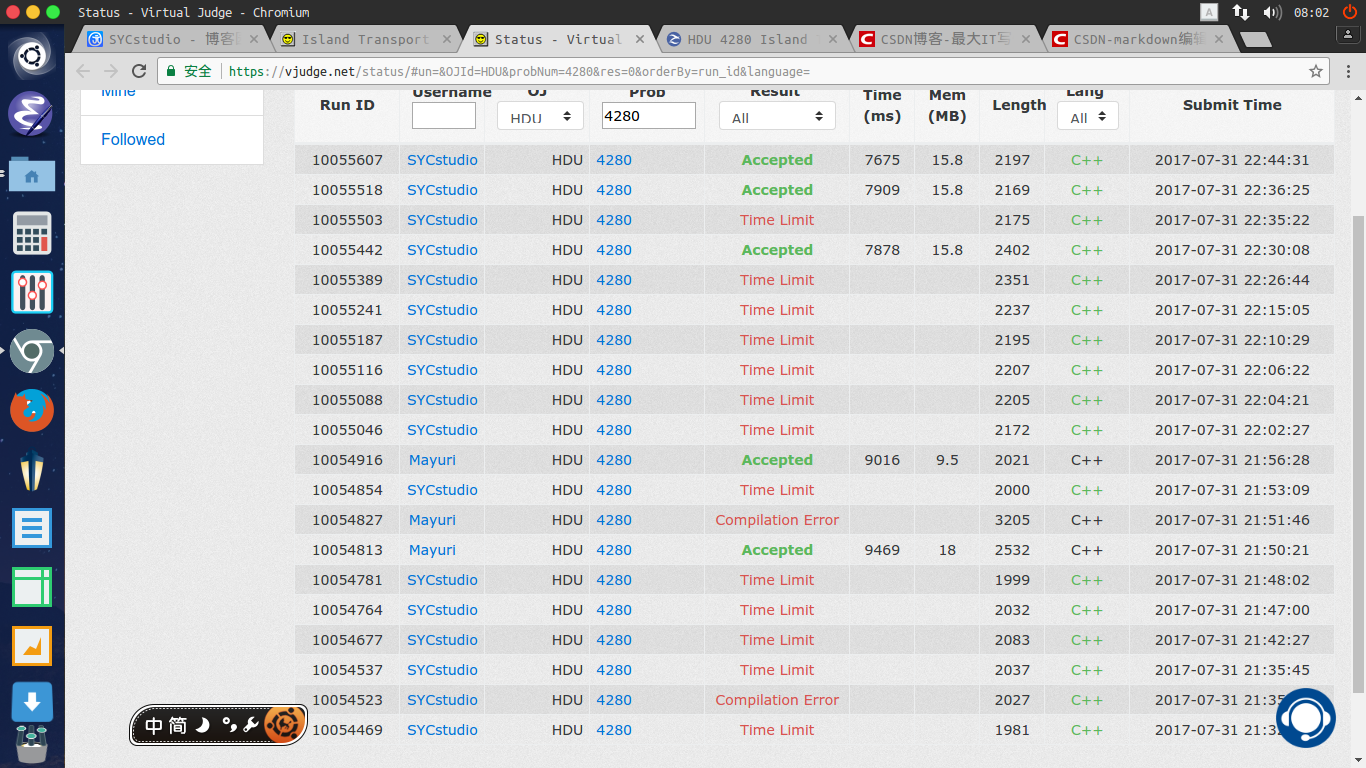# HDU 4280 Island Transport（网络流，最大流）

### Description

In the vast waters far far away, there are many islands. People are living on the islands, and all the transport among the islands relies on the ships.
You have a transportation company there. Some routes are opened for passengers. Each route is a straight line connecting two different islands, and it is bidirectional. Within an hour, a route can transport a certain number of passengers in one direction. For safety, no two routes are cross or overlap and no routes will pass an island except the departing island and the arriving island. Each island can be treated as a point on the XY plane coordinate system. X coordinate increase from west to east, and Y coordinate increase from south to north.
The transport capacity is important to you. Suppose many passengers depart from the westernmost island and would like to arrive at the easternmost island, the maximum number of passengers arrive at the latter within every hour is the transport capacity. Please calculate it.

### Input

The first line contains one integer T (1<=T<=20), the number of test cases.
Then T test cases follow. The first line of each test case contains two integers N and M (2<=N,M<=100000), the number of islands and the number of routes. Islands are number from 1 to N.
Then N lines follow. Each line contain two integers, the X and Y coordinate of an island. The K-th line in the N lines describes the island K. The absolute values of all the coordinates are no more than 100000.
Then M lines follow. Each line contains three integers I1, I2 (1<=I1,I2<=N) and C (1<=C<=10000) . It means there is a route connecting island I1 and island I2, and it can transport C passengers in one direction within an hour.
It is guaranteed that the routes obey the rules described above. There is only one island is westernmost and only one island is easternmost. No two islands would have the same coordinates. Each island can go to any other island by the routes.

### Output

For each test case, output an integer in one line, the transport capacity.

2
5 7
3 3
3 0
3 1
0 0
4 5
1 3 3
2 3 4
2 4 3
1 5 6
4 5 3
1 4 4
3 4 2
6 7
-1 -1
0 1
0 2
1 0
1 1
2 3
1 2 1
2 3 6
4 5 5
5 6 3
1 4 6
2 5 5
3 6 4

9
6

## 代码

#include<iostream>
#include<cstdio>
#include<cstdlib>
#include<cstring>
using namespace std;
//像这种卡时的题目尽量不要用STL
#define min(x,y) x>y? y : x
#define RG register

const int maxN=200031;//数组一定要开够
const int maxM=500031;
const int inf=2147483647;

struct Edge
{
int v,flow;
};

int n,m;
int S,T;
int cnt=-1;
int Next[maxM];
Edge E[maxM];
int cur[maxN];
int depth[maxN];
int Queue[maxN];

inline void Add_Edge(int u,int v,int flow);
int dfs(int u,int flow);

int main()
{
int Case;
while (Case--)//多组数据
{
cnt=-1;
int minx=inf,maxx=-inf;
for (int i=1;i<=n;i++)
{
if (x<minx)
{
minx=x;
S=i;
}
if (x>maxx)
{
maxx=x;
T=i;
}
}
for (int i=1;i<=m;i++)
{
}
int Ans=0;
while (1)
{
memset(depth,-1,sizeof(depth));//减少函数调用，把bfs放入主过程
int Top=1,Tail=0;
depth[S]=1;
Queue=S;
do
{
Tail++;
int u=Queue[Tail];
if (u==T)
break;
{
int v=E[i].v;
if ((E[i].flow>0)&&(depth[v]==-1))
{
depth[v]=depth[u]+1;
Top++;
Queue[Top]=v;
}
}
}
while (Top!=Tail);
if (depth[T]==-1)
break;
for (int i=1;i<=n;i++)//当前弧优化，这个优化力度很明显，一定要加

while (int di=dfs(S,inf))//dfs寻找增广路
Ans+=di;
}
printf("%d\n",Ans);
}
return 0;
}

{
int x=0;
scanf("%d",&x);//这里直接用scanf读入
return x;
int k=1;
char ch=getchar();
while (((ch>'9')||(ch<'0'))&&(ch!='-'))
ch=getchar();
if (ch=='-')
{
ch=getchar();
k=-1;
}
while ((ch>='0')&&(ch<='9'))
{
x=x*10+ch-48;
ch=getchar();
}
return x*k;
}

inline void Add_Edge(int u,int v,int flow)//添加边，注意正反向都是流量为flow，因为是双向边
{
cnt++;
E[cnt].v=v;
E[cnt].flow=flow;

cnt++;
E[cnt].v=u;
E[cnt].flow=flow;
return;
}

int dfs(int u,int flow)//dfs寻增广路
{
if (u==T)
return flow;
for (int &i=cur[u];i!=-1;i=Next[i])
{
int v=E[i].v;
if ((depth[v]==depth[u]+1)&&(E[i].flow>0))
{
int di=dfs(v,min(flow,E[i].flow));
if (di>0)
{
E[i].flow-=di;
E[i^1].flow+=di;
return di;
}
}
}
return 0;
}posted @ 2017-08-01 08:05  SYCstudio  阅读(...)  评论(...编辑  收藏